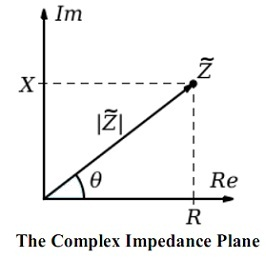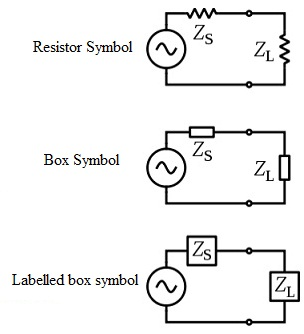#### Complex Impedances, Physics tutorial

Introduction:

Electrical impedance that you might simply refer to as impedance, explains a measure of opposition to alternating current (AC) and electrical impedance expands the concept of resistance to AC circuits, explaining not merely the relative amplitude units of the voltage and current, however as well the relative  phases. If a circuit is driven by direct current (DC) there is no difference between impedance and resistance; you might thus imagine it as the impedance having zero phase angle.

The symbol 'Z' is frequently utilized for impedance and it might be represented through writing its magnitude and phase in the form mod Z angle θ. You will though find out that, complex number representation of electrical impedance is more powerful for circuit analysis aims. The word impedance was first employed by Oliver Heaviside in July 1886 whereas Arthur Kennelly was the first to introduce impedance by complex numbers in the year 1893.

Impedance is stated as the frequency domain ratio of the voltage to the current. In another words, this is the voltage-current ratio for a single complex exponential at a particular frequency 'ω'. In common, impedance will be a complex number having the similar units as resistance, for which the SI unit is the ohm (Ω). For a sinusoidal current or voltage input, the polar form of the complex impedance associates the amplitude and phase of the voltage and current. In specific, the magnitude of the complex impedance is the ratio of the voltage amplitude to the current amplitude.

The phase of the complex impedance is the phase shift through which the current is ahead of the voltage.

The reciprocal of impedance is the admittance. Admittance is the current-to-voltage ratio, and it conventionally carries units of Siemens that was formerly termed as mhos.

Equivalent Impedance Transform:Impedance is symbolized as a complex quantity 'Z' and the word complex impedance might be used interchangeably; the polar form suitably captures both magnitude and phase features,

Z = |Z|e

Here, the magnitude |Z| represents the ratio of the voltage difference amplitude to the current amplitude, whereas the argument 'θ' gives the phase difference among voltage and current and j is the imaginary unit. In Cartesian form, in which the real part of impedance is the resistance and the imaginary portion is the reactance.

Here it is necessary for you to add or subtract impedances the Cartesian form is more suitable, however if quantities are multiplied or divided the calculation becomes simpler if the polar form is employed. A circuit computation, like finding the net impedance of two impedances in parallel, might need conversion between forms some times during the computation.

Electrical impedance and ohm's law:

The AC supply applying a voltage 'V' across a load 'Z' and driving a current 'I'. The meaning of electrical impedance can be comprehended by replacing it into Ohm's law.

V = I Z = I |Z|e

The magnitude of the impedance |Z| acts just similar to resistance, providing the drop in voltage amplitude across an impedance 'Z' for a given current 'I'.

The phase factor state us that the current lags the voltage through a phase of 'θ' (that is, in the time domain, the current signal is shifted θ/2π to the right by respect to the voltage signal).

Mere as impedance extends, the Ohm's law to cover AC circuits, other outcomes from DC circuit analysis like voltage division, current division, Norton's theorem and Thevenin's theorem, can as well be expanded  to AC circuits by means of substituting resistance by impedance.

Complex Voltage and Current:

We can sketch generalized impedances in a circuit having the similar symbol as a resistor or by a labeled box.

In order to make simpler your computations, it will be simpler for you to represent sinusoidal voltage and current waves as the complex-valued functions of time represented as V and I.V = |V| ej (ωt + ΦV)

I = |I| ej (ωt + ΦI)

If we define impedance as the ratio of such quantities

Z = V/I

And we replace such into Ohm's law and then we have:

|V| ej (ωt + ΦV) = |I| ej (ωt + ΦI) |Z| e

|V| ej(ωt + ΦV) = |I||Z|ej (ωt + ΦI +θ)

And it is noted that this should hold for all values of 't', we might equate the magnitudes and phases to get:

|V| = |I||Z|

Φv = ΦI

The magnitude equation is the well-known Ohm's law applied to the voltage and current amplitudes, whereas the second equation states the phase relationship.

Phasor:

A phasor is a constant complex number that you can generally deduce in exponential form, representing the complex amplitude (that is, magnitude and phase) of a sinusoidal function of time. Phasor are employed in the field of electrical engineering to simplify the computations comprising sinusoids, where they can frequently decrease a differential equation problem to an arithmetical one.

The impedance of a circuit element can be stated as the ratio of the phasor voltage across the element to the phasor current via the element, as found out by the relative amplitudes and phases of the current and voltage. This is similar to the statement from Ohm's law given above, identifying that the factors of ejωt cancel.

Device Specific Impedances

What follows beneath is the derivation of impedance for each of the three fundamental circuit elements, the resistor, the capacitor and the inductor.

However the idea can be expanded to state the relationship between the current and voltage of any random signal, such derivations will suppose sinusoidal signals, as any arbitrary signal can be approximated as the sum of sinusoids via Fourier analysis.

Resistor:

For a resistor, we encompass the relation:

vR(t) = iR(t)R

This is basically a statement of the Ohm's Law.

Considering the voltage signal to be:

vR(t) = Vp sin (ωt)

This follows that,

vR(t)/iR(t) = Vp sin (ωt)/Ip sin (ωt) = R

This states us that the ratio of AC voltage amplitude to AC current amplitude across the resistor is R, and that the AC voltage leads the AC current across a resistor by 0 degrees.

This outcome is generally deduced as:

Z resistor = R

Capacitor:

For a capacitor, we have the relationship:

iC(t) = C dvC(t)/dt

Let consider the voltage signal to be:

vC(t) = Vpsin(ωt)

This follows that:

dvC(t)/dt = ωVpcos(ωt)

And therefore,

vC(t)/iC(t) = Vpsin(ωt)/ωVpcos(ωt) = sin(ωt)/ωC sin(ωt + π/2)

This state us that the ratio of AC voltage amplitude to AC current amplitude across a capacitor is 1/ωC, and that the AC voltage leads the AC current across the capacitor by -90 degrees (or the AC current leads the AC voltage across the capacitor through 90 degrees).

This outcome is generally deduced in polar form, as:

Z capacitor = (1/ωC) e-jπ/2

Or, through applying the Euler's formula, as

Z capacitor = -j (1/ωC) = 1/jωC

Inductor:

For the inductor, we encompass the relation:

vL(t) = Ldi2L(t)/dt

This time, taking the current signal to be:

iL(t) = IPsin(ωt)

This follows that:

diL(t)/dt = ω IP cos(ωt)

And therefore,

vL(t)/iL(t) = ωIPLcos(ωt)/IPsin(ωt) = ωL sin (ωt + π/2)/sin (ωt)

This states us that the ratio of AC voltage amplitude to AC current amplitude across the inductor is ωL, and that the AC voltage leads the AC current across an inductor through 90 degrees.

S-plane impedance:

Impedance stated in terms of jω can severely only is applied to the circuits that are energized having a steady-state AC signal. The theory of impedance can be expanded to a circuit energized by any arbitrary signal by employing complex frequency rather than jω. The complex frequency is represented by the symbol  's' and is, in general, a complex number. Signals are deduced in terms of the complex frequency by taking the Laplace transform of the time domain expression of the signal. The impedance of the fundamental circuit elements in more general notation is as follows:

Element       Impedance expression

Resistor                     R

Inductor                    sL

Capacitor                 1/sC

For a DC circuit you can simplify this to s = 0. For a steady-state sinusoidal AC signal s = jω.

Combination of Impedances:

The net impedance of several simple networks of components can be computed by employing the rules for joining impedances in series and parallel. The rules are similar to those employed for joining resistances, apart from that the numbers in general will be complex numbers. In general case though, equivalent impedance transforms in addition to series and parallel will be needed.

Series combination:

If we join components in series, the current via each circuit element is similar; the net impedance is simply the sum of the component impedances.

Zeq = Z1 + Z2 + ...... + Zn

Parallel combination:

For components joined in parallel, the voltage across each and every circuit element is similar; the ratio of currents via any two elements is the inverse ratio of their impedances.

1/Zeq = 1/Z1 + 1/Z2 +.... + 1/Zn

Tutorsglobe: A way to secure high grade in your curriculum (Online Tutoring)

Expand your confidence, grow study skills and improve your grades.

Since 2009, Tutorsglobe has proactively helped millions of students to get better grades in school, college or university and score well in competitive tests with live, one-on-one online tutoring.

Using an advanced developed tutoring system providing little or no wait time, the students are connected on-demand with a tutor at www.tutorsglobe.com. Students work one-on-one, in real-time with a tutor, communicating and studying using a virtual whiteboard technology.  Scientific and mathematical notation, symbols, geometric figures, graphing and freehand drawing can be rendered quickly and easily in the advanced whiteboard.

Free to know our price and packages for online physics tutoring. Chat with us or submit request at info@tutorsglobe.com